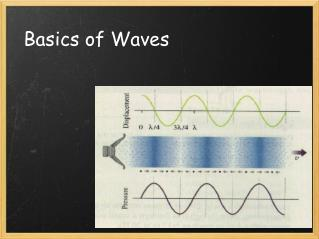DownloadDownload PresentationBasics of Waves

# Basics of Waves

Télécharger la présentation## Basics of Waves

- - - - - - - - - - - - - - - - - - - - - - - - - - - E N D - - - - - - - - - - - - - - - - - - - - - - - - - - -
##### Presentation Transcript

1. Basics of Waves

2. Properties of Waves: -Propagate through space -Cause Displacement Most Waves are Periodic - "Period" is the time it takes for a pattern to repeat ... - "Period" is the time it takes for a pattern to repeat (Har har)

3. Measurement of Waves Period  (Time for one cycle) Wavelength    (Space) λ Amplitude (displacement or power)

4. v = f x λ v is the wave velocity (the speed of sound in the case of sound waves). Units: m/s λ is the wavelength, or the length of space between one peak (maximum amplitude) and the next peak. Units: m (usually) f is "frequency" or the number of times something happens in a second. Units: Hz (Hertz) Danger Will Robeson! The speed of sound can change. How? this could also be measured from trough (the lowest amplitude) to trough New Unit alert! Hertz = 1/sec (per second)

5. Example Problem: Running Deer measures a sound wave with a frequency of 686 Hz. Using the speed of sound at room temperature (343 m/s), find the wavelength v=f ·λ v = 343 m/s f = 686 Hz λ = v/f = (343 m/s)/(686 Hz) = 0.5 m

6. f = 1/T T is the period (or total Time) that the wave takes to complete a cycle, or to repeat itself.  T is another of physic's overused letters. Many Physicists prefer to use the capital version of the Greek letter Tau... which basically looks like a T If each mark on the horizontal represents a second then the period of this wave is 2 seconds and the frequency is 0.5 Hz.

7. The speed of a "sound wavefront" (the speed that the peaks and troughs of a wave travel through space) can be calculated in the same way as other objects traveling through space: v = d/t Where v is the speed of sound through a material.  When you count the seconds between a flash of lightning (nearly instantaneous after a strike) and the sound of thunder many seconds later, you are finding the time for the sound wavefront to reach you. Tsunamis are giant sound wavefronts traveling through water (which can have a speed of sound up to almost five times that of air) until they hit a shoreline. Earthquakes also send sound waves through the core of the earth, which has helped scientist determine the composition of the inside of the earth. Ultrasound uses the same principle to create images based on materials having different speeds of sound.

8. Lea Tinning sees a flash of lightning hit lightning rod that she knows is 1 km away. It is a cold day, so Lea estimated that the speed of sound is 333 m/s. How long until she hears thunder? d = 1 km = 1000 m v = 333 m/s v = d/t t = d/v = (1000 m) / (333 m/s) = 3 seconds

9. Sound waves represent the compression and decompression of molecules or atoms. Since a vacuum, or "empty" space, lacks much of anything to compress or decompress, sound does not transmit under these conditions.  Peak Trough